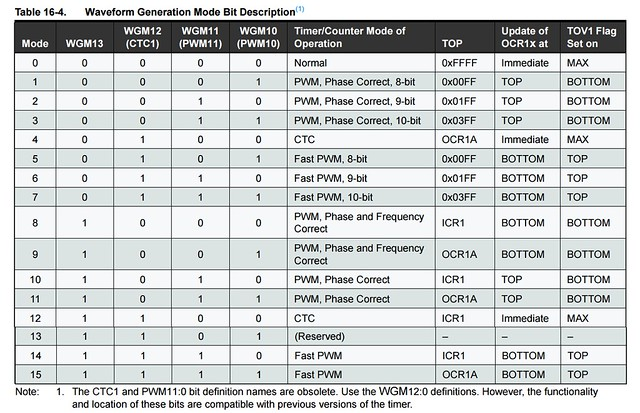# How to control a servomotor without arduino servo library

Please let us in the comment zone any suggestions that you think will improve the article!

If you like the article click the follow button  to stay in touch with us!

In the next project i intend to control a servomotor (a cheap one) directly from timers because with the library(servo library) i couldn’t obtained a real 180 degrees angle.

1. The theory about those servos:

A servomotor is actually an actuator with a dc motor, a gear box and some electronics to control the motor.

Basically, the electronics receive a repetitive signal and turn it into a position angle.

The most used frequency on those cheap servos is 50Hz(PWM) and 3 different duty cycles, 1ms, 1.5ms and 2ms – 1.5ms is for 0 degree (middle position), 1ms is 90 degree to the right and 2ms is for 90 degree to the left.

Now, my problem is that if i put the pwm in those duty cycles, the servo movement is smaller than 180 degree, so i am controling it directly with timers .

2. Controlling the servo without library

To see exactly what happens and how it reacts at the duty cycle i used a timer – more exactly, a timer 1 with phase correct pwm mode controlling the frequency from ICR1 register and duty cycle from OCR1A register (mode 10). To learn more about timer1 and how to control signals: http://www.eprojectszone.com/how-to-modify-the-pwm-frequency-on-the-arduino-part2timer-1-and-phase-correct-pwm-mode/With ICR1, we set the frequency and with OCR1A, we set the duty cycle on pin 9 (for pin 10 we could use OCR1B).

The formula for frequency is : f=fclk/(2*N*TOP), where TOP is defined by ICR1.

Now, to set 50 Hz, we need to know the TOP value so from the formula above TOP=20000, where N is 8 the prescaler.

Because the frequency of the signal is 50Hz, the time period is 20ms and ICR1=20000, so to have a 1ms duty cycle we must make the OCR1A=1000 for 1.5ms OCR1A=1500 and for 2000ms OCR1A=2000.

But, at those values my servo look something like this:

So, if you want a more precise control of the servo, those values are not good enough. With the program below you can set the OCR1A value to make your servo more precise.

void setup() {
pinMode(9, OUTPUT);
pinMode(10,OUTPUT);
TCCR1A=0;//reset the register
TCCR1B=0;//reset the register
TCNT1=0;
TCCR1A=0b10100010;//COM1A0,COM1B0 are 0, COM1A1, COM1B1 are 0 0
//also WGM10 are 0 and WGM11=1
TCCR1B=0b00010010;//WGM13 is 1 and WGM12 is 0 with prescaler equal 8
ICR1=20000;
OCR1A=2500;//here you set the values for duty cycle and also angle
//OCR1B=1000;//you can connect another servo here and control it
}
void loop() {
// put your main code here, to run repeatedly
}

For my servo, i used values for OCR1A=580, 1565 and 2550.